# The Chemistry of Acids and BasesPage 5

#### WATCH ALL SLIDES

K gives the ratio of ions (split up) to molecules (don’t split up)

HONORS ONLY!

Slide 44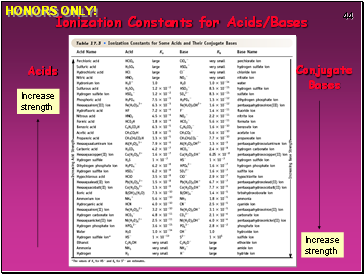Ionization Constants for Acids/Bases

Acids

Conjugate

Bases

Increase strength

Increase strength

HONORS ONLY!

Slide 45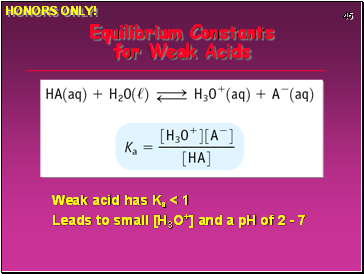Equilibrium Constants for Weak Acids

Weak acid has Ka < 1

Leads to small [H3O+] and a pH of 2 - 7

HONORS ONLY!

Slide 46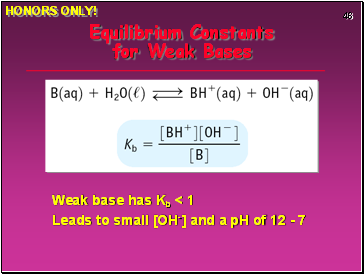Equilibrium Constants for Weak Bases

Weak base has Kb < 1

Leads to small [OH-] and a pH of 12 - 7

HONORS ONLY!

Slide 47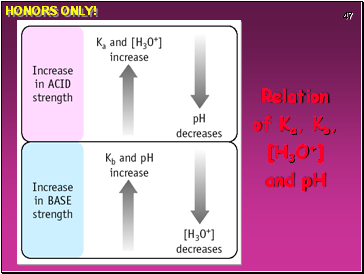Relation of Ka, Kb, [H3O+] and pH

HONORS ONLY!

Slide 48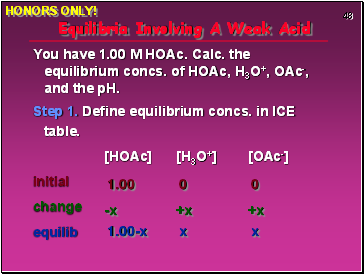Equilibria Involving A Weak Acid

You have 1.00 M HOAc. Calc. the equilibrium concs. of HOAc, H3O+, OAc-, and the pH.

Step 1. Define equilibrium concs. in ICE table.

[HOAc] [H3O+] [OAc-]

initial

change

equilib

1.00 0 0

-x +x +x

1.00-x x x

HONORS ONLY!

Slide 49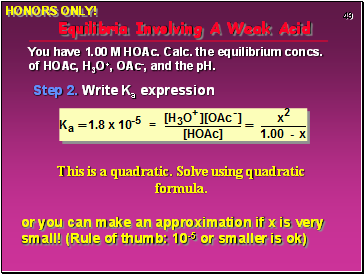Equilibria Involving A Weak Acid

Step 2. Write Ka expression

You have 1.00 M HOAc. Calc. the equilibrium concs. of HOAc, H3O+, OAc-, and the pH.

or you can make an approximation if x is very small! (Rule of thumb: 10-5 or smaller is ok)

HONORS ONLY!

Slide 50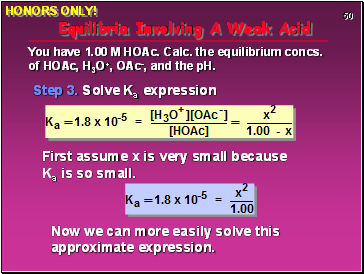Equilibria Involving A Weak Acid

Step 3. Solve Ka expression

You have 1.00 M HOAc. Calc. the equilibrium concs. of HOAc, H3O+, OAc-, and the pH.

First assume x is very small because Ka is so small.

Now we can more easily solve this approximate expression.

HONORS ONLY!

Slide 51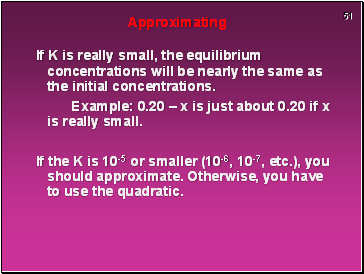Approximating

If K is really small, the equilibrium concentrations will be nearly the same as the initial concentrations.

Example: 0.20 – x is just about 0.20 if x is really small.

If the K is 10-5 or smaller (10-6, 10-7, etc.), you should approximate. Otherwise, you have to use the quadratic.

Slide 52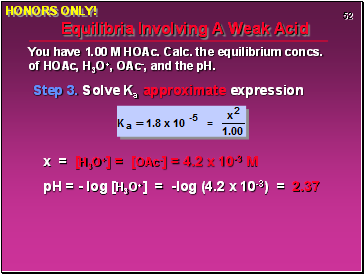Equilibria Involving A Weak Acid

Step 3. Solve Ka approximate expression

You have 1.00 M HOAc. Calc. the equilibrium concs. of HOAc, H3O+, OAc-, and the pH.

x = [H3O+] = [OAc-] = 4.2 x 10-3 M

pH = - log [H3O+] = -log (4.2 x 10-3) = 2.37

HONORS ONLY!

Slide 53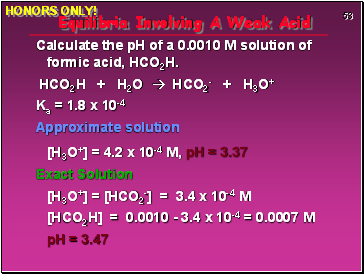Go to page:
1  2  3  4  5  6  7  8